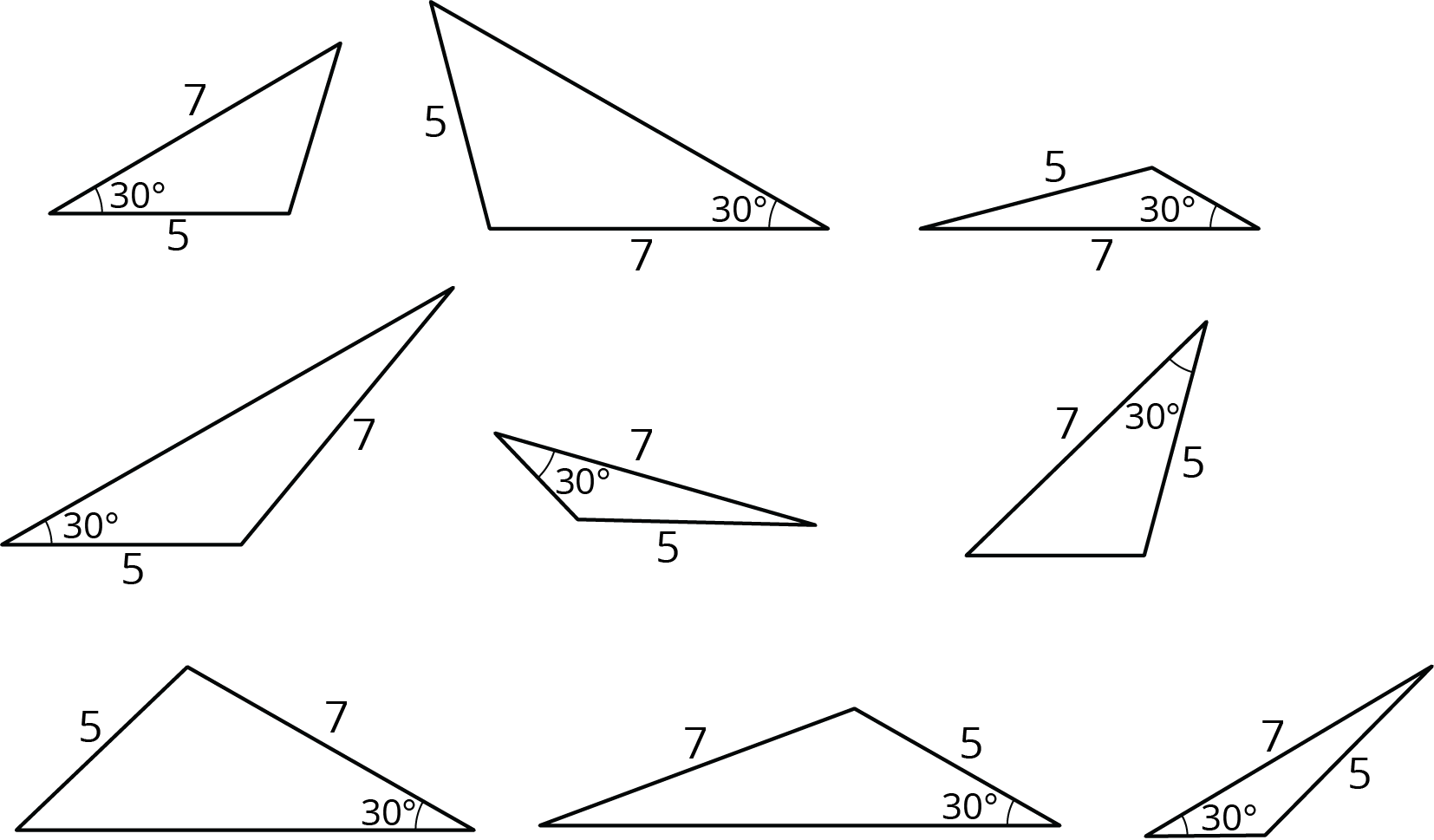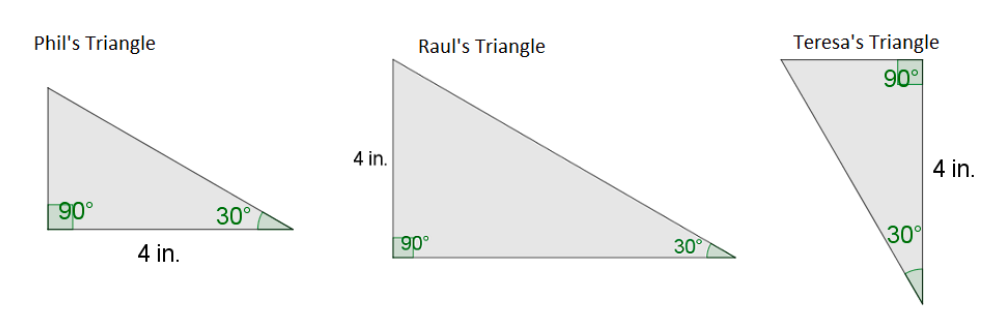# Geometry

## Objective

Identify unique and identical triangles.

## Common Core Standards

### Core Standards

?

• 7.G.A.2 — Draw (freehand, with ruler and protractor, and with technology) geometric shapes with given conditions. Focus on constructing triangles from three measures of angles or sides, noticing when the conditions determine a unique triangle, more than one triangle, or no triangle.

## Criteria for Success

?

1. Understand that the location and adjacency of angles and side lengths can determine identical or different triangles.
2. Identify identical copies of the same triangle.
3. Sketch drawings of the same triangle in different orientations.

## Tips for Teachers

?

In Lessons 14 and 15, students investigate the situations that create unique, more than one, or no triangle. In this lesson, students observe how the conditions described about a triangle can sometimes be represented in a diagram in different ways. They will begin to sketch triangles with given conditions and continue this in Lesson 15.

#### Fishtank Plus

• Problem Set
• Student Handout Editor
• Vocabulary Package

## Anchor Problems

?

### Problem 1

Examine this set of triangles.1. What is the same about the triangles in the set? What is different?
2. How many different triangles are there? Explain or show your reasoning.

#### References

Open Up Resources Grade 7 Unit 7Lesson 8.2 - 2 Sides and 1 Angle

### Problem 2

Phil, Raul, and Teresa each drew a right triangle with another angle measuring 30° and a side length of 4 inches. Their drawings are shown below (not drawn to scale).Phil claims that he drew the same triangle as Raul, but different from Teresa. Do you agree with Phil? Explain why or why not.

## Problem Set

?The following resources include problems and activities aligned to the objective of the lesson that can be used to create your own problem set.

• Include problems where students are given a set of triangles with the same measurements and must determine which triangles are the same but in different orientations and which triangles are different.